# What is DC Circuit Analysis?

What is DC Circuit Analysis?

DC circuit analysis is to understand and analyze DC circuits with ohm’s law, Kirchoff’s laws and similar methods. The fundamental relationship between voltage, current, and resistance in an electrical or electronic circuit is called Ohm’s Law.

All materials are made up of atoms, and all atoms are made up of protons, neutrons, and electrons. Protons have a positive electric charge. Neutrons have no electric charge (i.e. They are Neutral), while Electrons have a negative electric charge. Atoms are bound together by strong attractive forces that exist between the atomic nucleus and the electrons in its outer shell.

When these protons, neutrons and electrons are together inside the atom, they are happy and stable. But if we separate them, they want to reform and they start to exert an attraction potential called potential difference.

Now if we create a closed circuit, these loose electrons will start to move and will be dragged back to the protons creating a flow of electrons due to their attraction. This flow of electrons is called electric current. Electrons do not flow freely through the circuit as the material they pass through creates a restriction on the electron flow. This restriction is called resistance.

All basic electrical or electronic circuits, then, are made up of three separate but very related electrical quantities called Voltage ( v ), Current, ( i ) and Resistance ( Ω ).

Electrical Voltage
Voltage ( V ) is the potential energy of an electrical source stored in the form of an electrical charge. Voltage can be thought of as the force pushing electrons along a conductor, and the higher the voltage, the greater the ability to “push” electrons through a given circuit. Since energy is capable of doing work, this potential energy can be defined as the work required in joules to move electrons around a circuit from one point or node to another in the form of electric current.

Next, the voltage difference between any two points or junctions (called nodes) in a circuit is known as Potential Difference (pd) and is often referred to as Voltage Drop.

The potential difference between two points is measured in Volts, with the circuit symbol V or a lowercase “v”, although Energy, E with the lowercase letter “e” is sometimes used to denote an emf (electromotive force) produced. Then the greater the voltage, the greater the pressure (or thrust) and the greater the capacity to do work.

A constant voltage source is called DC Voltage, and a voltage whose voltage changes periodically over time is called AC voltage. Voltage is measured in volts; One volt is defined as the electrical pressure required to force an electrical current of one ampere through a resistor of one Ohm. Voltages are usually expressed in Volts, with prefixes used to denote lower multiples of voltage such as microvolts ( μV = 10 -6 V ), millivolts ( mV = 10 -3 V ) or kilovolts ( kV = 10 3 V ). The voltage can be positive or negative.

Batteries or power supplies are mostly used in electronic circuits and systems such as 5v, 12v, 24v etc. It is used to produce a constant DC (direct current) voltage source. AC (alternating current) voltage sources are available for domestic and industrial power and power transmission with lighting.

General electronics are powered by low voltage DC battery sources between 1.5V and 24V DC. The circuit symbol for a constant voltage source is usually given as a battery symbol with the plus, + and minus, – sign indicating the polarity direction. The circuit symbol for an alternating voltage source is a circle with a sine wave inside.

Voltage Symbols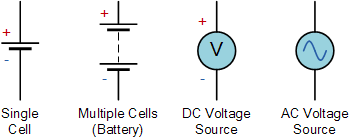A simple relationship can be established between a water tank and a voltage source. The higher the water tank is above the outlet, the higher the pressure of the water as more energy is released, the higher the voltage, the higher the potential energy as more electrons are released.

Voltage is always measured as the difference between any two points in a circuit, and the voltage between these two points is often referred to as “Voltage drop”. Note that voltage can exist in a circuit without current, but current cannot exist without any voltage source as it is voltage-free and therefore hates any short-circuit condition, whether DC or AC likes an open or semi-open circuit state.

Electrical current
Electric Current ( I ) is the movement or flow of electric charge and is measured in Amps with the symbol i by weight. It is a continuous and smooth flow (called drift) of electrons (negative particles of an atom) around a circuit that is “pushed” by a voltage source. In reality, electrons flow from the negative (–ve) terminal of the source to the positive (+ve) terminal, and for ease of understanding the circuit conventional current flow assumes that the current flows from the positive terminal to the negative terminal.

In circuit diagrams in general, the flow of current through the circuit includes an arrow, usually associated with the symbol I or a lowercase i, to indicate the true direction of the current. However, this arrow usually indicates the direction of conventional current flow, not the direction of actual flow.

Conventional Current Flow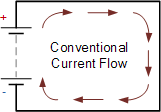Traditionally this is the flow of positive charge around a circuit from positive to negative. The diagram on the left shows the movement of positive charge (holes) around a closed circuit flowing from the positive pole of the power supply and returning to the negative pole of the power supply. This current flow from positive to negative is commonly known as conventional current flow.

This was the custom chosen during the discovery of electricity, where the direction of electric current is thought to flow in a circuit. To continue this line of thinking, the arrows shown in all circuit diagrams and diagrams, symbols for components such as diodes and transistors, indicate the conventional direction of current flow.

Then Conventional Current Flow gives the flow of electric current from positive to negative and in the opposite direction of true electron flow.

Electron Flow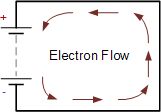The flow of electrons around the circuit is opposite to the direction of conventional current flow from negative to positive. The actual current flowing in an electrical circuit consists of electrons flowing through the negative pole (cathode) of the battery and returning.

This is because the charge on an electron is negative by definition and is therefore attracted to the positive terminal. This flow of electrons is called Electron Current Flow. Therefore, electrons actually flow around a circuit from the negative terminal to the positive.

Both conventional current flow and electron flow are used by many textbooks. In fact, it doesn’t matter which direction the current flows around the circuit as long as the direction is used consistently. The direction of current flow does not affect what the current does in the circuit. In general, conventional current flow is much easier to understand. From positive to negative.

In electronic circuits, the current source is given as a circle with an arrow showing its direction.

Current is measured in Amperes and amperage is defined as the number of electrons or charges ( Q in Coulombs ) ( t in seconds ) passing through a given point in the circuit in one second.

Electrical current is usually expressed in Amps, with prefixes used to denote microamperes (μA = 10 -6 A) or milliamperes (mA = 10 -3 A). Note that electric current can be positive in value or negative in value depending on the direction of flow around the circuit.

The current flowing in a single direction is called Direct Current or DC, and the current that changes back and forth in the circuit is called Alternating Current or AC. Whether AC or DC current flows through a circuit only when a voltage source is connected to it, its “flow” is limited by both the circuit’s resistance and the voltage source pushing it.

Also, since alternating currents (and voltages) are periodic and change over time, the “effective” or “RMS”, (Root Mean Square) value given as I rms produces the same average power loss equivalent to DC current Iaverage. Current sources are the opposite of voltage sources in that they like short or closed circuit conditions but dislike open circuit conditions because no current will flow.

Using the water tank relationship, the current is equivalent to the water flow through the pipe with the same flow through the pipe. The faster the water flow, the greater the current. Note that current cannot exist without voltage, so any current source DC or AC loves a short or semi-short circuit condition, but hates any open circuit condition because it prevents it from flowing.

Resistance
Resistance (R) is the capacity of a material to resist or impede the flow of current, or more specifically, the flow of electric charge in a circuit. The circuit element that does this perfectly is called a “Resistance”.

Resistance is a circuit element measured in Ohms, with prefixes used to denote Kilo-ohms ( kΩ = 10 3 Ω ) and Mega-ohms ( MΩ = 10 6 Ω ), represented by the Greek symbol ( Ω , Omega ). Note that the resistor is only positive and cannot be negative.

Resistance Symbols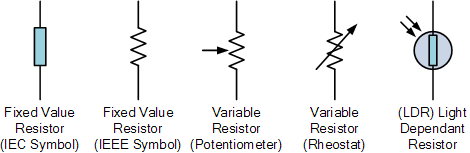The amount of resistance a resistor has is determined by the relationship of the current flowing through it to the voltage, which determines whether the circuit element is a “good conductor” – low resistance or “bad conductor” – high resistance. Low resistance e.g. 1Ω or less means the circuit is a good conductor made of materials like copper, aluminum or carbon, while resistance of 1MΩ or higher means the circuit is a bad conductor made of insulating materials like glass, porcelain.

On the other hand, a “semiconductor” such as silicon or germanium is a material whose resistance is halfway between a good conductor and a good insulator. Hence the name “semiconductor”. Semiconductors Diode and Transistor etc. used to make.

Resistance may be non-linear in nature, but never negative. Linear resistance obeys Ohm’s Law as the voltage across the resistor is linearly proportional to the current flowing through it. The non-linear resistor does not obey Ohm’s Law but has a voltage drop across it proportional to some strength of the current.

The resistor is pure and since the AC impedance of a resistor is equal to the DC resistance it is not affected by frequency and cannot be negative as a result. Remember that resistance is always positive and never negative.

A resistor is classified as a passive circuit element and therefore cannot supply power or store energy. Instead, resistors absorb power, which appears as heat and light. The power in a resistor is always positive regardless of voltage polarity and current direction.

For very low resistance values, e.g. milli-ohms, (mΩ) it is sometimes much easier to use the inverse of the resistor (1/R) rather than the resistor (R) itself. The equivalent of resistance is called Conductivity and represents the ability of a conductor or device to conduct electricity.

High conductivity values ​​mean a good conductor like copper, while low conductivity values ​​mean a bad conductor like wood. The standard unit of measurement for conductivity is the Siemens symbol ( S ).

The unit used for conductivity is the mho (ohm written backwards), symbolized by an inverted Ohm sign ℧. Power can also be expressed using conductivity as: P = i 2 /G = v 2 G .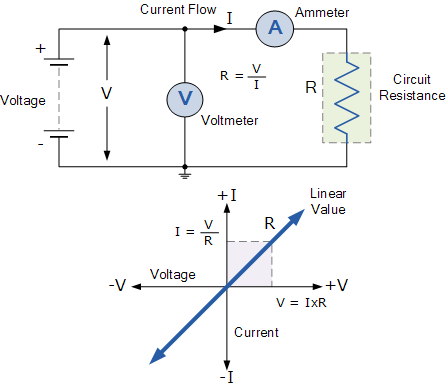İn a nutshell
In a fixed resistance linear circuit, if we increase the voltage, the current will increase, and similarly, if we decrease the voltage, the current will decrease. This means that if the voltage is high, the current is high, and if the voltage is low, the current is low.

Likewise, for a given voltage, if we increase the resistance, the current will decrease, and if we decrease the resistance, the current will increase. This means that if the resistance is high, the current is low, and if the resistance is low, the current is high.

Then we can see that the current flow around a circuit is directly proportional to voltage ( ∝ ), causing V↑ I↑, but inversely proportional to resistance ( 1/∝ ), causing R↑ I↓ .

A basic summary of the three issues is given below.

• Voltage or potential difference is the measure of potential energy between two points in a circuit and is often referred to as “voltage drop”.
• When a voltage source is connected to a closed loop circuit, the voltage will produce a current flowing around the circuit.
• In DC voltage sources, the symbols +ve (positive) and -ve (negative) are used to indicate the polarity of the voltage source.
• Voltage is measured in Volts and has the symbol V for voltage or E for electrical energy.
• Current flow is the combination of electron flow and hole flow through a circuit.
• Current is the continuous and uniform flow of charge around the circuit and is measured in Amps or Amps and has the symbol I.
• Current is directly proportional to Voltage ( I ∝ V )
• The effective (rms) value of alternating current has the average power loss equivalent to direct current flowing through a resistive element.
• Resistance is the resistance to current flowing around a circuit.
• Low resistance values ​​mean a conductor and high resistance values ​​mean an insulator.
• Current is inversely proportional to resistance ( I 1/∝ R )
• Resistance is measured in Ohms and has the Greek symbol Ω or the letter R.

In our next content on DC Circuits, we’ll look at Ohm’s Law, a mathematical equation that explains the relationship between Voltage, Current, and Resistance in electrical circuits and is the foundation of electronics and electrical engineering. Ohm’s Law is defined as: V = I*R .